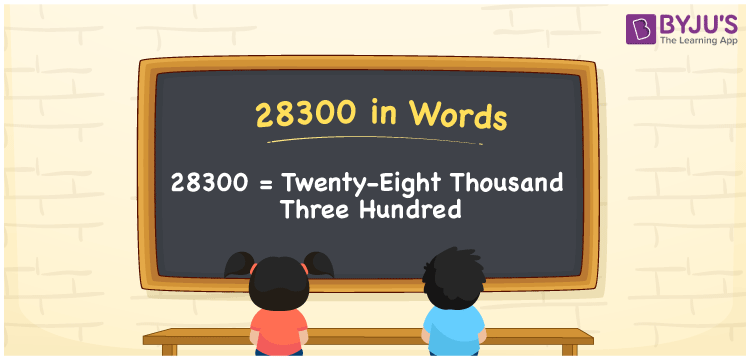# 28300 in Words

We can write 28300 in words as Twenty-eight thousand three hundred. If you saved Rs. 28300 in January, you can say, “I saved Twenty-eight thousand three hundred rupees in the month of January”. Similarly, we can express different quantities or measures that are equivalent to 28300 using the word form, i.e., Twenty-eight thousand three hundred. Let’s understand how to convert the cardinal number 28300 into words using a place value chart and some facts about 28300.

 28300 in words Twenty-eight thousand three hundred Twenty-eight thousand three hundred in Numbers 28300

## 28300 in English words

Generally, we express numbers in words using the English alphabet. Therefore, we can spell 28300 in English as “Twenty-eight thousand three hundred”.## How to Write 28300 in Words?

Writing a number in word form is simple when we use the place value chart. The below table represents the five-column place value chart as the number 28300 has five digits.

 Ten thousand Thousands Hundreds Tens Ones 2 8 3 0 0

Here, ones = 0, tens = 0, hundred = 3, thousands = 8, ten thousands = 2

Let us expand these digits according to their place values.

2 × Ten thousand + 8 × Thousand + 3 × Hundred + 0 × Ten + 0 × One

= 2 × 10000 + 8 × 1000 + 3 × 100 + 0 × 10 + 0 × 1

= 20000 + 8000 + 300

= Twenty thousand + Eight thousand + Three hundred

= Twenty-eight thousand three hundred

Therefore, 28300 in words = Twenty-eight thousand three hundred.

As we know, 28300 is a natural number that is the successor of 28299 and the predecessor of 28301.

28300 in words – Twenty-eight thousand three hundred

Is 28300 an odd number? – No

Is 28300 an even number? – Yes

Is 28300 a perfect square number? – No

Is 28300 a perfect cube number? – No

Is 28300 a prime number? – No

Is 28300 a composite number? – Yes

## Frequently Asked Questions on 28300 in Words

Q1

### How do you write an amount Rs. 28300 in words?

We can write an amount Rs. 28300 in words as “Twenty-eight thousand three hundred rupees”.
Q2

### What is 28300 in word form?

The word form of 28300 is Twenty-eight thousand three hundred.
Q3

### Find the value of Twenty-eight thousand three hundred minus Twenty-five thousand.

Twenty-eight thousand three hundred minus Twenty-five thousand = 28300 – 25000 = 3300, i.e. Three thousand three hundred.